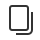• 介绍
• 设计
• 开发
• 数据
• 运营
• 第三方平台
• 社区# 数据监听器

## # 使用数据监听器``````Component({
properties: {
numberA: {
type: Number,
value: 0
},
numberB: {
type: Number,
value: 0
}
},
attached: function() {
this.setData({
numberA: 1,
numberB: 2
})
},
observers: {
'numberA, numberB': function (numberA, numberB) {
// 在 numberA 或者 numberB 被设置时，执行这个函数
this.setData({
sum: numberA + numberB
})
}
}
});
``````

## # 监听字段语法``````Component({
observers: {
'some.subfield': function(subfield) {
// 使用 setData 设置 this.data.some.subfield 时触发
// （除此以外，使用 setData 设置 this.data.some 也会触发）
subfield === this.data.some.subfield
},
'arr': function(arr12) {
// 使用 setData 设置 this.data.arr 时触发
// （除此以外，使用 setData 设置 this.data.arr 也会触发）
arr12 === this.data.arr
},
}
});
````````````Component({
observers: {
'some.field.**': function(field) {
// 使用 setData 设置 this.data.some.field 本身或其下任何子数据字段时触发
// （除此以外，使用 setData 设置 this.data.some 也会触发）
field === this.data.some.field
},
},
data: {
some: {
field: {
subfield: [1, 2, 3]
}
}
},
attached: function() {
// 会触发 observers
this.setData({
'some.field': {}
});

// 会触发 observers
// this.setData({
//     'some.field.xxx': {}
// });

// 会触发 observers
// this.setData({
//     'some': {}
// });
}
});

````````````Component({
observers: {
'**': function(value) {
// 数据发生变化即触发，这里的 value 是 properties 和 data 的合集
}
}
})
``````

### # Bugs & Tips

• 数据监听器监听的是`setData`中涉及的数据字段，并且只有这些字段发生改变时才会触发，类似`observer`;
• 在数据监听器中再次进行`setData`设置被监听的字段，可能会造成循环监听，请注意使用；
• 由于小程序对`setData`进行了优化操作，所以如果同步的多次设置`setData`那么实际上之后执行最后一次`setData`引起的`observers```````Component({
properties: {
numberA: {
type: Number,
value: 0
},
numberB: {
type: Number,
value: 0
}
},
attached: function() {
this.setData({
numberA: 1,
numberB: 2
});
this.setData({
numberA: 2,
numberB: 3
})
},
observers: {
'numberA, numberB': function (numberA, numberB) {
// 在 numberA 或者 numberB 被设置时，执行这个函数
this.setData({
sum: numberA + numberB
})
}
}
});
``````